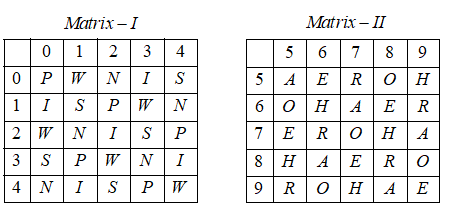A word is represented by only one set of numbers as given in anyone of the alternatives. The set of numbers given in the alternatives are represented by two classes of alphabets as in the two matrices given below. The columns and rows of Matrix I are numbered from 0 to 4 and that of Matrix II from 5 to 9. A letter can be represented first by its row and next by its column number. e.g, ‘N’ can be represented by 02, 21, etc. ‘O’ can be represented by 65, 96 etc. Similarly, you have to identify the correct set for the word given in question.        SORROW

# A word is represented by only one set of numbers as given in anyone of the alternatives. The set of numbers given in the alternatives are represented by two classes of alphabets as in the two matrices given below. The columns and rows of Matrix I are numbered from 0 to 4 and that of Matrix II from 5 to 9. A letter can be represented first by its row and next by its column number. e.g, ‘N’ can be represented by 02, 21, etc. ‘O’ can be represented by 65, 96 etc. Similarly, you have to identify the correct set for the word given in question.SORROW

1. A

23, 86, 69, 88, 65, 33

2. B

23, 43, 14, 33, 65, 78

3. C

11, 66, 69, 65, 59, 97

4. D

42, 65, 95, 88, 77, 44

Fill Out the Form for Expert Academic Guidance!l

+91

Live ClassesBooksTest SeriesSelf Learning

Verify OTP Code (required)

### Solution:+91

Live ClassesBooksTest SeriesSelf Learning

Verify OTP Code (required)